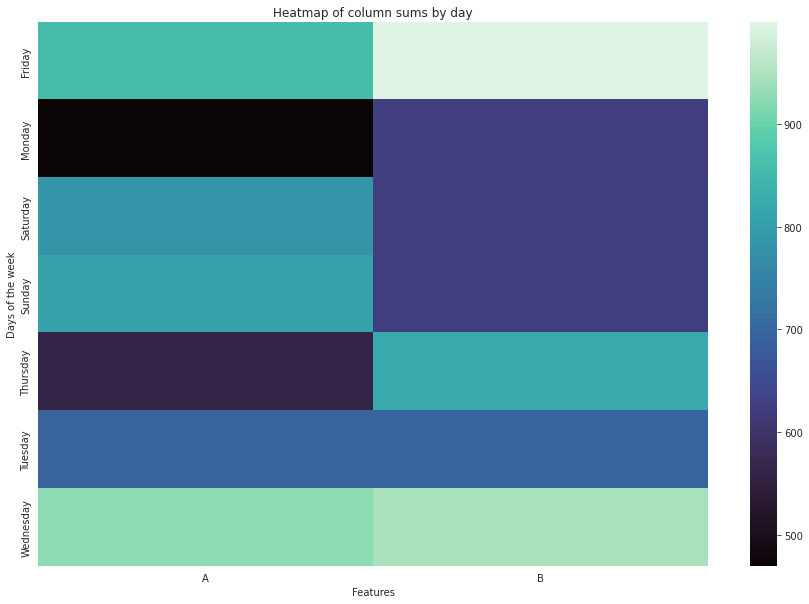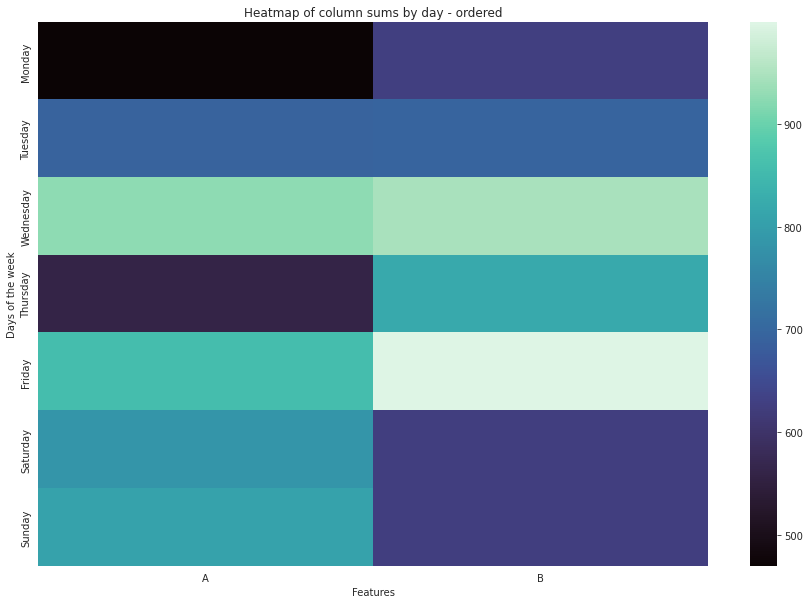# Explicit ordering for categorical data in Python

## Utilise the Pandas functionality for more human-friendly outputsCategorical data with Pandas. Image by author.

This is a quick reference post covering the topic of explicit ordering for categorical data in Pandas. The underlying assumption here is the data one is working with is: a) categorical b) has an explicit order.

# What is categorical data in Pandas?

In statistics, categorical data is data that can be divided into groups. The groups are usually based on a certain characteristic of the data, such as color, shape, or size.

Categorical data is often used in Python to help organise information. For example, if you were working with a dataset of people’s names, you could use categorical data to group the names by gender.

# Creating categorical data from a dataset

There are a number of ways of creating categorical data using your data. A few options are:

1. Create categories from continuous data. For example a column contains ages (continuous data) into age brackets.
2. Convert discrete data points (e.g. months of the year, cities) into categories

In our example, we will be focusing of the second point, and using days of the week as categorical data.

# Why order categorical data

Categorical data is often seen as unordered, but there is value in assigning an order to the categories. When working with categorical data in pandas, the order of the categories can be important for:

• visualisations: makes more sense to us human when reading and interpreting
• computations: faster computation when doing calculations
• memory: data formatted as categories can lead to significant reduction in memory usage

Let’s look at a practical example. If we plot the output from a dataset containing days of week (as objects data type) we can see the categories (days of the week) are ordered in an unintiuitve way:Heatmap with unordered y-axis. Image by author.

Working through this example, we can organise and visualise this better.

# How to format and order categorical data

In an earlier post, I covered how to translate text using Python and Google Translate while staying in your chosen development/lab environment. I will be extending the examples used there.

For the sake of ease, here is the complete code from the previous example to generate some random data:

There are a few different ways to define the order of categories in pandas. The simplest way is to use the CategoricalDtype constructor. Here’s the generic code:

`import pandas as pdfrom pandas.api.types import CategoricalDtypecategory_values = [‘A’, ‘B’, ‘C’]category_order = CategoricalDtype(category_values, ordered=True)df['category_field']= df['category_field'].astype(category_order)`

Applying this to our example and producing a heatmap with Seaborn. Here we can see the variation of the data based on the day order we dedfined.Heatmap with ordered categorical data. Image by author.

And here’s the code to produce the above:

# Resources

1. Pandas documentation for Categorical data: https://pandas.pydata.org/docs/reference/api/pandas.Categorical.html

--

--## Abhinav Saraswat

A wearer of many hats. Interested in NLP, geospatial, time series, financial modelling, game design